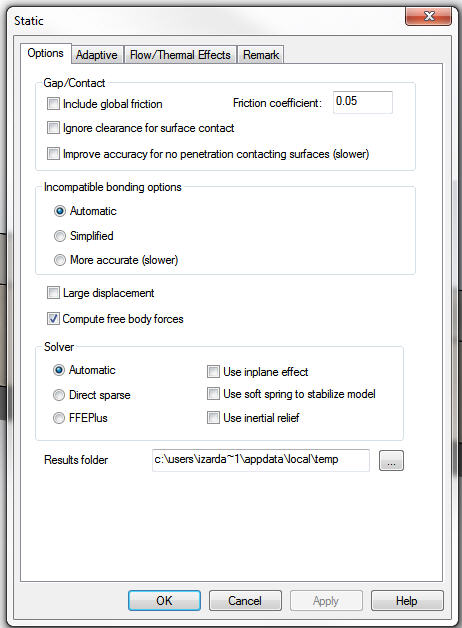# Which Solver? FFEPlus vs. Direct Sparse – PART 1Article by Irfan Zardadkhan, PhD, CSWE updated January 15, 2013

###### Article

SolidWorks simulation provides two options to solve the set of FEA algebraic equations; iterative or direct solution methods.

The iterative solver, FFEPlus, uses approximate techniques to solve the problem. It assumes a solution and then calculates the associated errors. The iterations continue until the errors become acceptable.

The direct solver, Direct Sparse, solves the set of equations directly without any approximations and hence there are no errors associated with the solution process. There will still be discretization errors which are present both in iterative or direct solvers.

A solver may be selected changing the study properties from the study feature tree.Solver Options

So the question is, which solver should we select. Since the direct sparse solver uses no approximations during the solution phase, it might seem like an obvious choice. Here is a comparison of the solution time of the two solvers for various degrees of freedom.

This study makes use of a simple cantilevered beam with load applied on the free end. Since there a no non-linearities, we see a steady growth in solution time with the increase in DoF when using an interative solver. The gradient is much more steeper when using the direct solver. In fact, the study with 1.1 million DoF was aborted after no solution was found after 30 minutes of running with the direct solver. So for cases with no non-linearities and large DoF, it is best to use the iterative solver rather than the direct solver. The direct solver requires far more memory than the iterative solver and as we approach the limit of the available physical memory, the solution  becomes extremely large. So when do we use the direct solver? A following post will address that question.

#### Get Certified SOLIDWORKS Services from Javelin